## Topic: Counting

Free Printable Worksheets on Counting

## Count the Objects – Counting 0 through 20This worksheet is from our book, “Mastering the Math Milestone” for kindergartners. It’s from the chapter on counting numbers 0 through 20....

## Counting Numbers Up to 100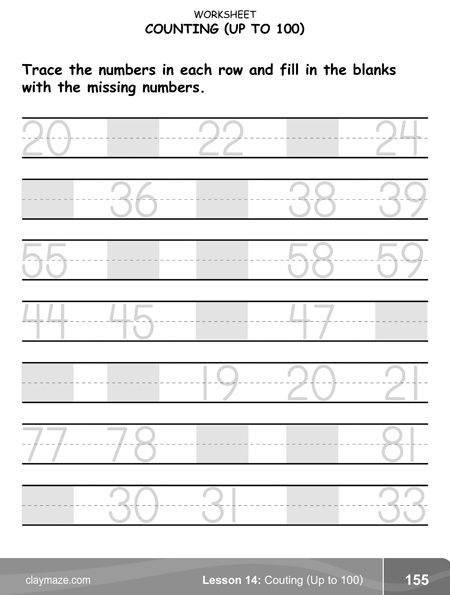This counting worksheet is from the book, “Mastering the Math Milestone“. This worksheet is for practice in writing, tracing and counting the numbers through 100....

## Counting Tally MarksThis worksheet on tallying is from our book, “Mastering the Math Milestone“. This is for practice in counting and totaling tally marks in a chart. It is also an introduction to...

## Tally the Objects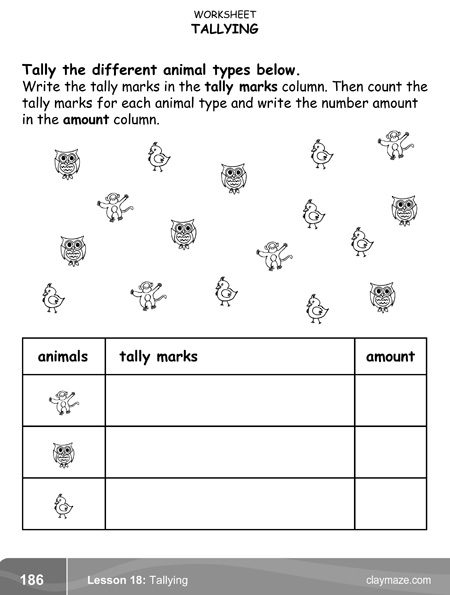This worksheet is from the tallying section of our book, “Mastering the Math Milestone“. On this worksheet, the child will fill out the chart by tallying each different type of animal...

## Read and Write Numbers (0 through 10)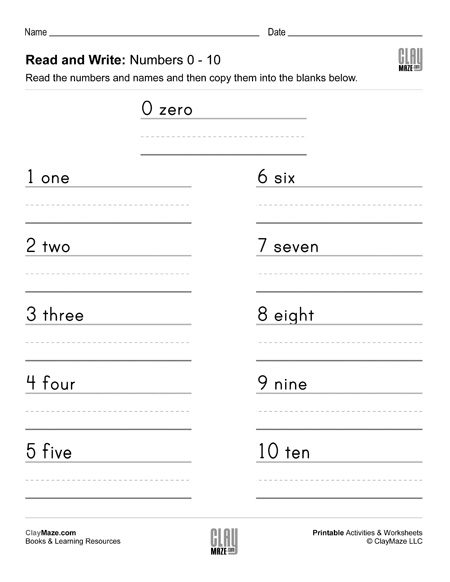Worksheet for children learning how to spell and write the numbers 0 through 10. Download the free, printable worksheet....

## Read and Write Numbers (0 to 100 by 10’s)Download our worksheet for number writing practice – numbers 0 through 100, counting by 10’s. This worksheet is for spelling and writing numbers and also helps in learning to count up...

## Read and Write Numbers (11 through 20)Number and number word writing practice worksheet for the numbers 11 through 20. This worksheet is recommended for children learning to write and spell numbers....

## Roman Numerals Reference ChartA reference chart of useful Roman numerals and their corresponding Arabic numerals. Download and print our Roman numeral worksheets: Arabic Numerals to Roman Numerals: Lower Numbers Arabic...

## Arabic Numerals to Roman Numerals: Lower Numbers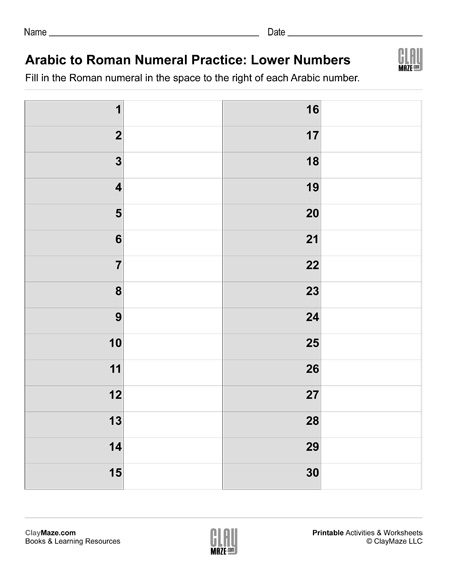Roman numerals free practice worksheet. Convert the Arabic numerals to Roman Numerals. – lower numbers Click here for our Roman numeral reference chart....

## Arabic Numerals to Roman Numerals: Higher NumbersFree worksheet on Roman numerals. Write the Roman numerals for each of the given Arabic numerals. – higher numbers Click here for our Roman numeral reference chart....

## Roman Numerals to Arabic Numerals: Lower Numbers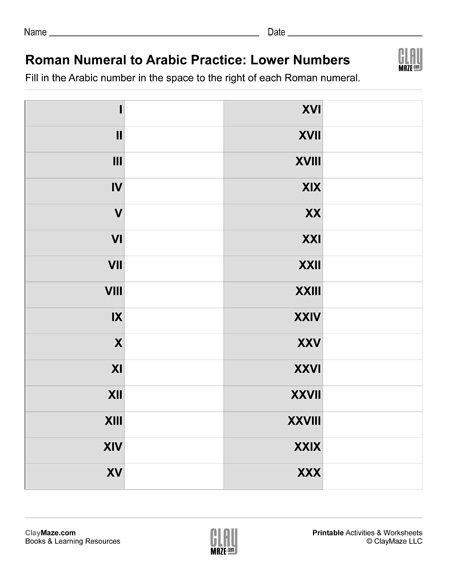Free Roman numerals worksheet. Write the Arabic numerals for each of the given Roman numerals. – lower numbers Click here for our Roman numeral reference chart....

## Roman Numerals to Arabic Numerals: Higher NumbersRoman numerals free practice worksheet. Convert the Roman numerals to Arabic Numerals. – higher numbers Click here for our Roman numeral reference chart....

## Basic Statistics: Probability I (Set 1)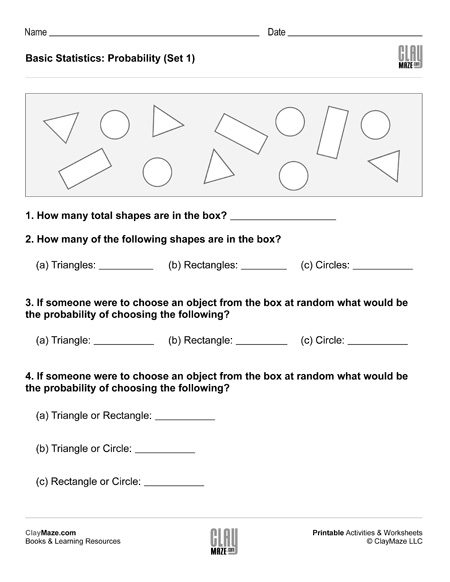This statistics worksheet is on basic probability. There are a number of different shapes in the box and the student is asked to find the probabilities of selecting the different shapes at random....

## Basic Statistics: Probability I (Set 2)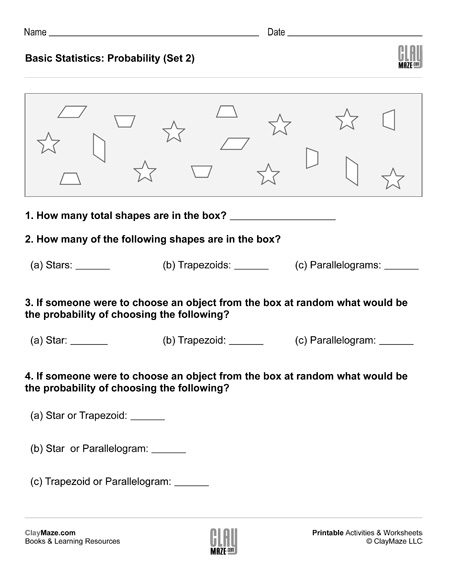This free worksheet covers basic probability. The student is asked several questions about the number of shapes in the box. The student can then use those values to find the probabilities of...

## Basic Statistics: Probability I (Set 3)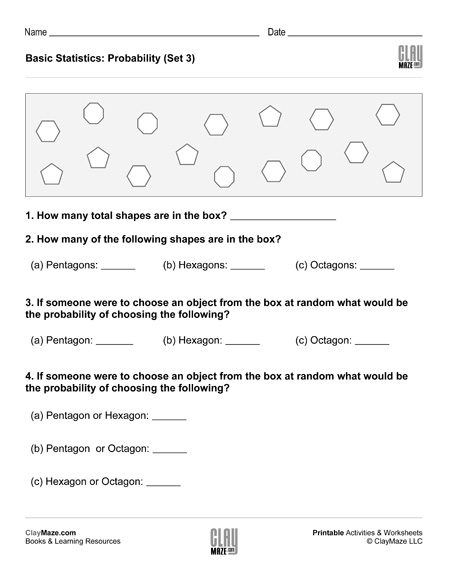This is a basic probability worksheet. The questions are based on the number of shapes in the box and the student is asked to find the probabilities of randomly choosing the different shapes....